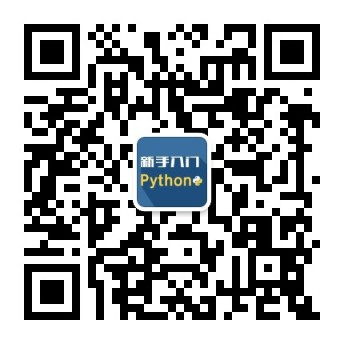2022
08-13

# c语言如何进行异或？1不用第三变量交换两个数

```A = A ^ B;
B = A ^ B;
A = A ^ B;```

```

A = A ^ B;   .............(1)
B = A ^ B;   .............(2)
A = A ^ B;   .............(3)

(2) => B = (A ^ B) ^  B  => B = A
(3) => A = (A ^ B) ^ (A) => A = B

```

2固定位翻转

```

0 ^ 1 = 1  1 ^ 1 = 0

```

3不用条件语句实现整数绝对值

```

A ^ 0xFF.. = ~A
A ^ 0      =  A

```